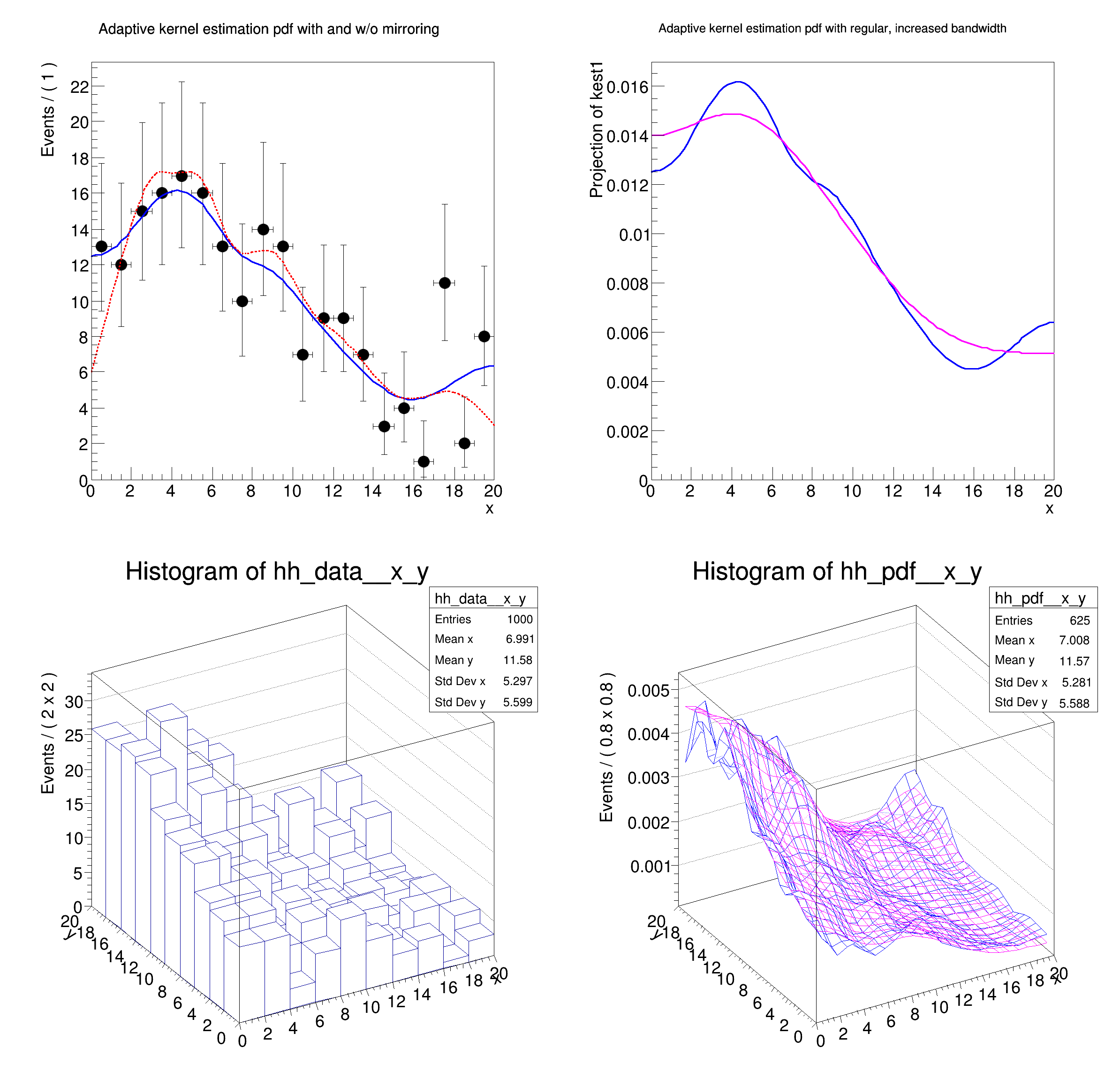ROOT   Reference Guiderf707_kernelestimation.C File Reference

## Detailed Description

Special p.d.f.'s: using non-parametric (multi-dimensional) kernel estimation p.d.f.s␛[1mRooFit v3.60 -- Developed by Wouter Verkerke and David Kirkby␛[0m
Copyright (C) 2000-2013 NIKHEF, University of California & Stanford University
#include "RooRealVar.h"
#include "RooDataSet.h"
#include "RooGaussian.h"
#include "RooConstVar.h"
#include "RooPolynomial.h"
#include "RooKeysPdf.h"
#include "RooNDKeysPdf.h"
#include "RooProdPdf.h"
#include "TCanvas.h"
#include "TAxis.h"
#include "TH1.h"
#include "RooPlot.h"
using namespace RooFit;
{
// C r e a t e l o w s t a t s 1 - D d a t a s e t
// -------------------------------------------------------
// Create a toy pdf for sampling
RooRealVar x("x", "x", 0, 20);
RooPolynomial p("p", "p", x, RooArgList(RooConst(0.01), RooConst(-0.01), RooConst(0.0004)));
// Sample 500 events from p
RooDataSet *data1 = p.generate(x, 200);
// C r e a t e 1 - D k e r n e l e s t i m a t i o n p d f
// ---------------------------------------------------------------
// Create adaptive kernel estimation pdf. In this configuration the input data
// is mirrored over the boundaries to minimize edge effects in distribution
// that do not fall to zero towards the edges
RooKeysPdf kest1("kest1", "kest1", x, *data1, RooKeysPdf::MirrorBoth);
// An adaptive kernel estimation pdf on the same data without mirroring option
// for comparison
RooKeysPdf kest2("kest2", "kest2", x, *data1, RooKeysPdf::NoMirror);
// Adaptive kernel estimation pdf with increased bandwidth scale factor
// (promotes smoothness over detail preservation)
RooKeysPdf kest3("kest1", "kest1", x, *data1, RooKeysPdf::MirrorBoth, 2);
// Plot kernel estimation pdfs with and without mirroring over data
RooPlot *frame = x.frame(Title("Adaptive kernel estimation pdf with and w/o mirroring"), Bins(20));
data1->plotOn(frame);
kest1.plotOn(frame);
kest2.plotOn(frame, LineStyle(kDashed), LineColor(kRed));
// Plot kernel estimation pdfs with regular and increased bandwidth
RooPlot *frame2 = x.frame(Title("Adaptive kernel estimation pdf with regular, increased bandwidth"));
kest1.plotOn(frame2);
kest3.plotOn(frame2, LineColor(kMagenta));
// C r e a t e l o w s t a t s 2 - D d a t a s e t
// -------------------------------------------------------
// Construct a 2D toy pdf for sampling
RooRealVar y("y", "y", 0, 20);
RooPolynomial py("py", "py", y, RooArgList(RooConst(0.01), RooConst(0.01), RooConst(-0.0004)));
RooProdPdf pxy("pxy", "pxy", RooArgSet(p, py));
RooDataSet *data2 = pxy.generate(RooArgSet(x, y), 1000);
// C r e a t e 2 - D k e r n e l e s t i m a t i o n p d f
// ---------------------------------------------------------------
// Create 2D adaptive kernel estimation pdf with mirroring
RooNDKeysPdf kest4("kest4", "kest4", RooArgSet(x, y), *data2, "am");
// Create 2D adaptive kernel estimation pdf with mirroring and double bandwidth
RooNDKeysPdf kest5("kest5", "kest5", RooArgSet(x, y), *data2, "am", 2);
// Create a histogram of the data
TH1 *hh_data = data2->createHistogram("hh_data", x, Binning(10), YVar(y, Binning(10)));
// Create histogram of the 2d kernel estimation pdfs
TH1 *hh_pdf = kest4.createHistogram("hh_pdf", x, Binning(25), YVar(y, Binning(25)));
TH1 *hh_pdf2 = kest5.createHistogram("hh_pdf2", x, Binning(25), YVar(y, Binning(25)));
hh_pdf->SetLineColor(kBlue);
TCanvas *c = new TCanvas("rf707_kernelestimation", "rf707_kernelestimation", 800, 800);
c->Divide(2, 2);
c->cd(1);
frame->GetYaxis()->SetTitleOffset(1.4);
frame->Draw();
c->cd(2);
frame2->GetYaxis()->SetTitleOffset(1.8);
frame2->Draw();
c->cd(3);
hh_data->GetZaxis()->SetTitleOffset(1.4);
hh_data->Draw("lego");
c->cd(4);
hh_pdf->GetZaxis()->SetTitleOffset(2.4);
hh_pdf->Draw("surf");
hh_pdf2->Draw("surfsame");
}
#define c(i)
Definition: RSha256.hxx:101
@ kRed
Definition: Rtypes.h:64
@ kMagenta
Definition: Rtypes.h:64
@ kBlue
Definition: Rtypes.h:64
@ kDashed
Definition: TAttLine.h:48
virtual RooPlot * plotOn(RooPlot *frame, const RooCmdArg &arg1=RooCmdArg::none(), const RooCmdArg &arg2=RooCmdArg::none(), const RooCmdArg &arg3=RooCmdArg::none(), const RooCmdArg &arg4=RooCmdArg::none(), const RooCmdArg &arg5=RooCmdArg::none(), const RooCmdArg &arg6=RooCmdArg::none(), const RooCmdArg &arg7=RooCmdArg::none(), const RooCmdArg &arg8=RooCmdArg::none()) const
Calls RooPlot* plotOn(RooPlot* frame, const RooLinkedList& cmdList) const ;.
Definition: RooAbsData.cxx:546
RooArgList is a container object that can hold multiple RooAbsArg objects.
Definition: RooArgList.h:21
RooArgSet is a container object that can hold multiple RooAbsArg objects.
Definition: RooArgSet.h:28
RooDataSet is a container class to hold unbinned data.
Definition: RooDataSet.h:33
TH2F * createHistogram(const RooAbsRealLValue &var1, const RooAbsRealLValue &var2, const char *cuts="", const char *name="hist") const
Create a TH2F histogram of the distribution of the specified variable using this dataset.
Class RooKeysPdf implements a one-dimensional kernel estimation p.d.f which model the distribution of...
Definition: RooKeysPdf.h:25
Generic N-dimensional implementation of a kernel estimation p.d.f.
Definition: RooNDKeysPdf.h:48
A RooPlot is a plot frame and a container for graphics objects within that frame.
Definition: RooPlot.h:44
TAxis * GetYaxis() const
Definition: RooPlot.cxx:1277
virtual void Draw(Option_t *options=0)
Draw this plot and all of the elements it contains.
Definition: RooPlot.cxx:712
RooPolynomial implements a polynomial p.d.f of the form.
Definition: RooPolynomial.h:28
RooProdPdf is an efficient implementation of a product of PDFs of the form.
Definition: RooProdPdf.h:31
RooRealVar represents a variable that can be changed from the outside.
Definition: RooRealVar.h:35
virtual void SetTitleOffset(Float_t offset=1)
Set distance between the axis and the axis title.
Definition: TAttAxis.cxx:294
virtual void SetLineColor(Color_t lcolor)
Set the line color.
Definition: TAttLine.h:40
The Canvas class.
Definition: TCanvas.h:27
The TH1 histogram class.
Definition: TH1.h:56
TAxis * GetZaxis()
Definition: TH1.h:318
virtual void Draw(Option_t *option="")
Draw this histogram with options.
Definition: TH1.cxx:2998
RooCmdArg YVar(const RooAbsRealLValue &var, const RooCmdArg &arg=RooCmdArg::none())
RooConstVar & RooConst(Double_t val)
RooCmdArg Bins(Int_t nbin)
RooCmdArg Binning(const RooAbsBinning &binning)
RooCmdArg LineColor(Color_t color)
RooCmdArg LineStyle(Style_t style)
Double_t y[n]
Definition: legend1.C:17
Double_t x[n]
Definition: legend1.C:17
The namespace RooFit contains mostly switches that change the behaviour of functions of PDFs (or othe...
const char * Title
Definition: TXMLSetup.cxx:67
Date
07/2008

Definition in file rf707_kernelestimation.C.